# Class 8 RD Sharma Solutions- Chapter 18 Practical Geometry – Exercise 18.1

• Last Updated : 06 May, 2021

### Question 1: Construct a quadrilateral ABCD in which AB = 4.4 cm, BC = 4 cm, CD = 6.4 cm, DA = 3.8 cm and BD = 6.6 cm?

Solution:

Given: AB = 4.4 cm, BC = 4 cm, CD = 6.4 cm, DA = 3.8 cm and BD = 6.6 cm.

Attention reader! All those who say programming isn't for kids, just haven't met the right mentors yet. Join the  Demo Class for First Step to Coding Coursespecifically designed for students of class 8 to 12.

The students will get to learn more about the world of programming in these free classes which will definitely help them in making a wise career choice in the future.

Let’s divide the quadrilateral into two triangles,

i.e., ΔABD and ΔBCD

Steps for the construction of a quadrilateral:

Step 1: Applying SSS congruency rule, Draw line BD of length 6.6 cm.

Step 2: Now, cut an arc with B as the centre and radius BC = 4 cm. Similarly by taking D as centre and radius CD = 6.4 cm.

Step 3: Join the intersection point from B and D and mark it as C.

Step 4: Now for vertex A, cut an arc by taking B as the centre and radius BA = 4.4 cm. Similarly by taking D as centre and radius DA = 3.8 cm.

Step 5: Join the intersection point from B and D and mark it as A.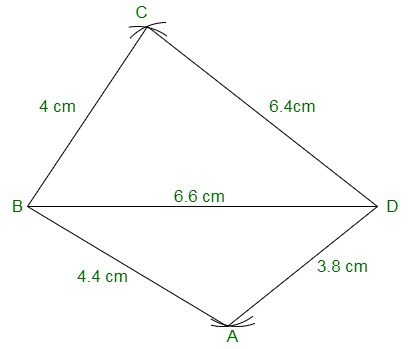### Question 2: Construct a quadrilateral ABCD in which AB = BC = 5.5 cm, CD = 4 cm, DA = 6.3 cm, AC = 9.4 cm Measure BD?

Solution:

Given: AB = BC = 5.5 cm, CD = 4 cm, DA = 6.3 cm, AC = 9.4 cm

We have to measure BD.

Steps for construction of the quadrilateral:

Step 1: Draw a line segment AB = 5.5 cm

Step 2: With B as centre and radius BC = 5.5 cm cut an arc. Mark the intersection point as C.

Step 3: With A as centre and radius AC = 9.4 cm cut an arc to intersect at point C.

Step 4: With C as centre and radius CD = 4 cm cut an arc. Mark the intersection point as D.

Step 5: With A as centre and radius AD = 6.3 cm cut an arc to intersect at point D.

Step 6: Now join BC, CD, and AD

Hence, the Measure of BD is 5.1 cm.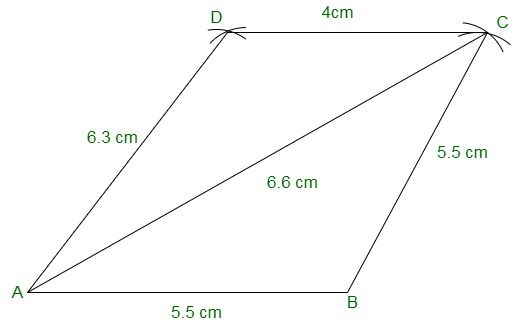### Question 3: Construct a quadrilateral XYZW in which XY = 5 cm, YZ = 6 cm, ZW = 7 cm, WX = 3 cm and XZ = 9 cm?

Solution:

We have, XY = 5cm, YZ = 6cm, ZW = 7cm, WX = 3cm and XZ = 9cm.

Step 1: Draw a line segment XZ with length = 9 cm.

Step 2: Taking X as the centre mark an arc of radius XY = 5 cm. Taking Z as centre and radius ZY = 6 cm, repeat the procedure.

Step 3: Mark the intersection point of X and Z as Y.

Step 4: For vertex W, taking X as the centre mark an arc of radius XW = 3 cm. Taking Z as centre and radius ZW = 7 cm, repeat the procedure.

Step 5: Mark the intersection point of X and Z as W.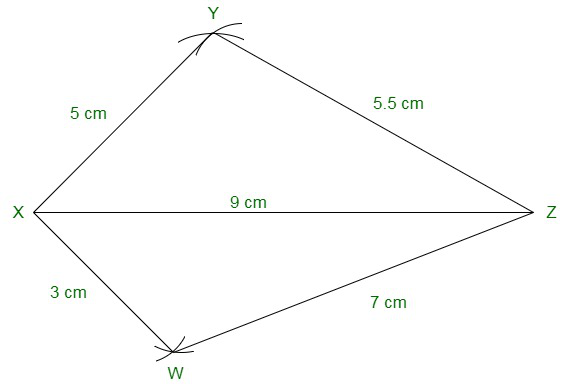### Question 4: Construct a parallelogram PQRS such that PQ = 5.2 cm, PR = 6.8 cm, and QS = 8.2 cm?

Solution:

We have, PQ = 5.2 cm, PR = 6.8 cm, and QS = 8.2 cm.

Steps to construct a parallelogram:

Step 1: Draw a line segment QS of length = 8.2 cm.

Step 2: Divide the line segment QS into two equal parts such that each one is = 4.1 cm. Mark the midpoint as O.

Step 3: Taking O as the centre, draw arcs of length 3.4 cm each on both the sides of the points O. Mark these points as P and R respectively

Step 4: Taking Q as a centre and radius QR = 5.2cm, draw an arc that intersects point R.

Step 5: Also, taking Q as a centre and radius QP = 5.2cm, again draw an arc that intersects point P.

Step 6: Join sides PQ, PS, QR, and RS to form a parallelogram.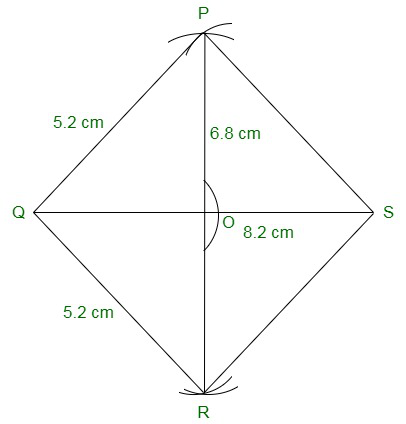### Question 5: Construct a rhombus with a side of 6 cm and one diagonal of 8 cm. Measure the other diagonal?

Solution:

In a rhombus, all the sides of a rhombus are equal and diagonals bisect each other.

We have a side 6 cm and one diagonal 8 cm.

Steps to construct a rhombus:

Step 1: Draw a line segment XZ of length 8 cm.

Step 2: Taking a radius = 6 cm, cut an arc with X as the centre. Repeat the procedure with Z as the centre and radius = 6 cm.

Step 3: Mark the intersection point of X and Z as Y.

Step 4: Taking a radius = 6 cm, cut an arc with W as the centre. Repeat the procedure with Z as the centre and radius = 6 cm.

Step 5: Mark the intersection point of X and Z as W.

Step 6: Join the line segments XY, XW, XZ, and ZY to form a rhombus.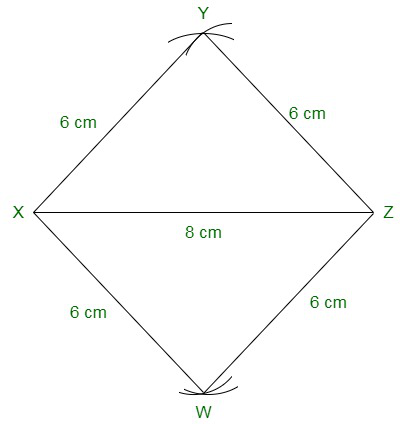### Question 6: Construct a kite ABCD in which AB = 4 cm, BC = 4.9 cm, AC = 7.2 cm?

Solution:

We have, AB = 4 cm, BC = 4.9 cm, AC = 7.2 cm.

Steps to construct a kite:

Step 1: Draw a line segment AC with length =  7.2 cm.

Step 2: Taking a radius of 4 cm and cut an arc by taking A as the centre. Also, taking C as the centre, repeat the procedure with radius = 4.9 cm.

Step 3: Label the intersection point of A and C and mark it as B.

Step 4: Considering vertex D, draw an arc with A as the centre. Also, taking C as the centre, repeat the procedure with radius = 4.9 cm.

Step 5: Label the intersection point of A and C and mark it as D.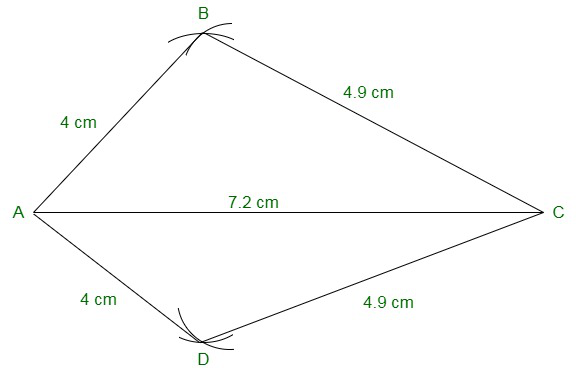### Question 7: Construct, if possible, a quadrilateral ABCD given AB = 6 cm, BC = 3.7 cm, CD = 5.7 cm, AD = 5.5 cm and BD = 6.1 cm. Give reasons for not being able to construct it, if you cannot?

Solution:

We have, AB = 6 cm, BC = 3.7 cm, CD = 5.7 cm, AD = 5.5 cm and BD = 6.1 cm.

Step 1: Draw a line segment AB with length = 6 cm.

Step 2: Taking A as the centre, draw an arc of a radius of 5.5 cm. Mark this point as D.

Step 3: Taking B as a centre, draw an arc of a radius of 6.1 cm intersecting point D.

Step 4: Also, with B as a centre, draw another arc of a radius of 3.7 cm. Mark this point as C.

Step 5: Taking D as a centre, draw an arc of a radius of 5.7 cm intersecting point C.

Step 6: Join the line segments, AD, BD, BC, and DC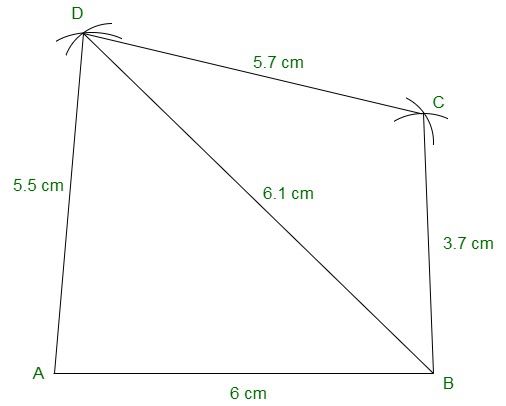### Question 8: Construct, if possible, a quadrilateral ABCD in which AB = 6 cm, BC = 7 cm, CD = 3 cm, AD = 5.5 cm and AC = 11 cm. Give reasons for not being able to construct, if you cannot. (Not possible, because in triangle ACD, AD + CD<AC)?

Solution:

We have, AB = 6 cm, BC = 7 cm, CD = 3 cm, AD = 5.5 cm and AC = 11 cm.

Since, we know, In any triangle the sum of the length of its two sides must be greater than that of the third side, therefore a quadrilateral with such dimensions cannot be constructed.

Considering in triangle ACD,

AD + CD = 5.5 + 3 = 8.5 cm

Given, AC = 11 cm

Now, AD + CD < AC which is not possible.

Hence, the construction cannot be performed.

My Personal Notes arrow_drop_up